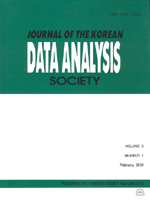상세검색
최근 검색어 전체 삭제
다국어입력
즐겨찾기0KCI등재 학술저널

# A Note on a Test Procedure under the Proportional Hazards Model

• 등재여부 : KCI등재
• 2016.12
• 2889 - 2896 (8 pages)

In this paper, we investigate some properties of a test procedure, which may be used whether two survival or hazard functions coincide or not under the proportional hazards assumption. Also we discuss that this proposed test can be applied for detecting some specific values of the treatment effect with comparison to the control group. For this purpose, first of all, we review the construction of the test statistic and testing procedure in some detail. Then we comment briefly on the consistency of the test by reasoning that the limiting power tends to unity under the general alternatives. Also we derive the limiting power of test under the scheme of the contiguous alternatives with some appropriate assumptions and the conditions which are known as the Noethter s condition. Then we illustrate the test procedure with a numerical example to test the equality of two underlying distributions. Finally we discuss some interesting aspects related with the proportional hazards model and corresponding tests.

1. Introduction

2. Review of Test Procedure

3. Asymptotic Properties under Contiguous Alternative

4. An Example and Some Concluding Remarks

References